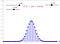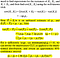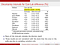# Comparing two proportions in the same survey

## When can we be confident in a difference of two proportions?

Statistics courses often deal with the problem of two independent proportions. Researchers conduct two surveys or experiments, which are independent of one another. The calculation is for proportions in the two independent samples. The question is: are the two proportions different?

This article returns to the problem of comparing two proportions in the same survey.

# The key differences

A YouGov survey question asks who “would make the best Prime Minister”. There are four options:

• Boris Johnson;
• Keir Starmer;
• Not sure;
• Refused.

Could Starmer’s lead be due to the random error that surveys have?

The traditional problem relates to proportions in independent samples. Researchers might wish to know what proportion of people select an option. Those two surveys could be at different times, or for different populations.

Our problem is distinct: in the same survey question, do proportions in options differ?

Now, the proportions are not independent. The proportions for all options must sum to 100%. The random chance of one proportion fluctuating affects all the others.

# Margins of sampling error for differences

Statistical thinking is more important than statistical arithmetic.

Sampling error is the random error from having a sample, rather than the whole population.

The common refrain is of ‘plus or minus three points’. That figure has three assumptions:

• The true proportion is 50%. Sampling error is lower for smaller proportions.
• The confidence level is 95%. Higher confidence levels means wider intervals.
• It is a simple random sample with 1,000 respondents.

A reader may look at the margin of sampling error for a survey estimate. That margin applies to support for an individual response option. It does not apply to leads.

Why is the margin of sampling error wider for party or candidate leads?

There is a range around each estimate: it could be somewhat higher or lower. By taking the difference of two uncertain estimates, that range is even greater. If the Labour share is too high by chance, the Conservative share is likely to be too low for the same reason.

# Following the arithmetic

Imagine you ask people about their preferred colour: Red or Blue. Every respondent goes through the same trial: picking Red or Blue. Since the survey is the sum of its respondents, those trials sum together.

The number of people picking Red follows a Binomial distribution.If the true proportion was 0.5, and the sample was 50: we would get 29/50 in 6% of samples. (Image: Malin Christersson)

Rather than calculate those figures, we can approximate with the Normal distribution. In general, this approximation is good enough.

It depends on the Central Limit Theorem. The larger the sample, the more ‘Normal’ the sampling distribution appears.

Suppose people could pick from three colours: Red, Blue or Green. Our interest is the difference between the Red and Blue shares.

The Red proportion affects the Blue share. We want to understand how much these two proportions co-vary.

As the Red proportion goes up, how much does the Blue share go down? Correlation is a normalised version of covariance.

The number of people choosing each colour choices follows a Multinomial distribution. That distribution has interesting properties:

• The share of Red and the share of Blue are both Binomial distributions;
• The sum of the Red and Blue shares is also a Binomial distribution.

Thanks to those properties, the covariance is quick to calculate. From there, we can get an approximate confidence interval for the difference:That is a good trick. (Image: The American Statistician/Scott and Seber)

That approximation is to a multivariate Normal distribution. In general again, this is a good approximation. There are certain conditions, such as small samples, where this is not appropriate.

The YouGov article states:

With Starmer now on 35% to Johnson’s 31%, not only is this the Labour leader’s strongest showing yet, it is also YouGov’s first lead for Starmer that is outside the margin of error.

The survey was of 1,652 adults in Great Britain. Responses were from 18th to 19th August 2020.

Assume this poll is a simple random sample. At 95% confidence, the margin of sampling error calculation is:

• Starmer share: 2.3%

Their central estimate of Starmer’s lead is 4 points. The lead has a plausible range from 0 to 8 points.

In their data tables, YouGov round the estimates. For the given values, the plausible range appears to be wholly above zero.

That seems to be the basis for saying the lead is “outside the margin of error”.

Is it reasonable to assume we have a simple random sample?

Simple random samples mean everyone in the population had equal chance of selection. Vote intention and leader preference polls will not have that sampling method. This assumption is a polite fiction for internet panel polls.

We could seek to account for a survey design’s complexity. The design factor is the ratio between standard errors of a simple sample and a complex survey. Design factors can vary between different questions in the same survey.

Ipsos MORI’s polling for RAJAR has a national design factor of 1.6. NatCen’s British Social Attitudes 2018 survey has design factors of around 1.3.

If we assume a uniform design factor of 1.5, the calculation becomes:

• Starmer share: 3.5%

Starmer’s lead has a plausible range from -2 to 10 points. That is under a less stringent assumption than a simple random sample (design factor of 1).

House effects can mean one company shows higher figures for politicians and parties. Being close to the polling average indicates centrality, not accuracy.

It remains important to highlight assumptions which underpin these calculations.

There are no golden roads in statistics. There are different ways to answer the same question. We could pursue an exact calculation, rather than an multivariate Normal approximation.The actual General Election result in 2015 was outside all the intervals. (Image: LSE/Kuha and Sturgis)

We could resample the survey responses, again and again. We use those new samples to construct a bootstrapped confidence interval. Prof Kuha and Prof Sturgis (LSE) suggest this approach.

Whatever the chosen method, uncertainty matters when analysing survey estimates.

Sampling variation is one way that survey estimates can deviate from population parameters. There are many types of non-sampling errors too, which are harder to quantify.

This blog looks at the use of statistics in Britain and beyond. It is written by RSS Statistical Ambassador and Chartered Statistician @anthonybmasters.

## More from Anthony B. Masters

This blog looks at the use of statistics in Britain and beyond. It is written by RSS Statistical Ambassador and Chartered Statistician @anthonybmasters.